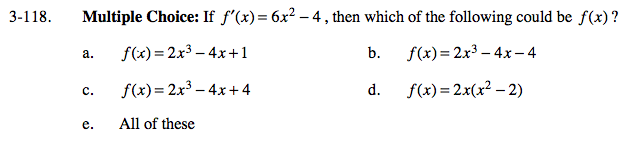### Home > CALC > Chapter 3 > Lesson 3.3.3 > Problem3-118

3-118.
1. Choice: If f ′(x) = 6x2 − 4, then which of the following could be f(x)? Homework Help ✎

1. f(x) = 2x3 − 4x + l

2. f(x) = 2x3 − 4x − 4

3. f(x) = 2x3 − 4x + 4

4. f(x) = 2x(x2 − 2)

5. All of theseWork backwards. Find the derivative of each distractor.